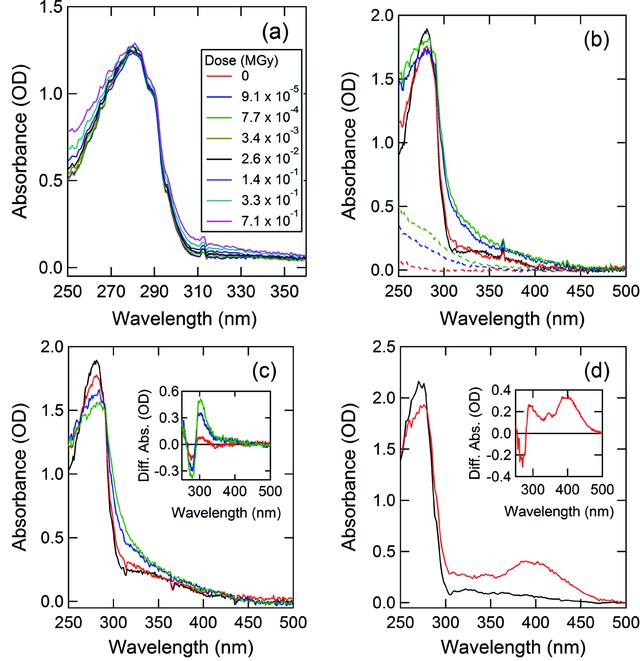disable zoom     view article Figure 4 Absorption spectra of sample crystals measured before and after X-ray irradiation. (a), (b), (c) are results for glucose isomerase, and (d) is a result for thaumatin. (a) Absorption spectra measured under the condition at low doses. The dose was increased stepwise. (b) High-dose measurements were performed on the crystal (solid line) and the cryo-solution (broken line). Black, red, blue and green lines indicate absorbed doses of 0, 2, 20 and 40 MGy, respectively. (c) The net absorption spectra calculated by subtracting the absorbance of the cryo-solution from that of the crystal. The colors indicate the same dose conditions as in (b). The inset figure shows the difference spectra obtained by subtracting the absorbance at 0 MGy from the absorbances at 2, 20 and 40 MGy. (d) Absorption spectrum after subtracting the absorbance of the cryo-solution from that of the thaumatin crystal. Black and red indicate absorbed doses of 0 and 2.7 MGy, respectively. The inset figure shows the difference spectra obtained by subtracting the absorbance at 0 MGy from the absorbance at 2.7 MGy.JOURNAL OFSYNCHROTRONRADIATION
ISSN: 1600-5775
Volume 20| Part 6| October 2013| Pages 948-952